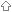Last update January 17, 2008

Error Messages / Compiler Errors's.length' is not an lvalueConflicting SymbolsA struct is not a valid initializer for an array's.length' is not an lvalueProblem code:```void main() { char[] s; s.length++; }```

Change`s.length++;`
to`s.length = s.length + 1;`Conflicting SymbolsProblem code:```import std.ctype; import std.string; void main() { char[] s = "some string"; s = toupper(s); printf("%.*s\n", s); }```

Error message:```c:\dmd\bin\..\src\phobos\std\string.d(502): function toupper conflicts with ctype.toupper at c:\dmd\bin\..\src\phobos\std\ctype.d(36)```

There are multiple solutions. The simplest is to change` s = toupper(s);`
to` s = std.string.toupper(s);`A struct is not a valid initializer for an arrayProblem code:`int[] data = { 17, 23, 42, 69, 105, 666 };`

Arrays are initialized using square brackets []`int[] data = [ 17, 23, 42, 69, 105, 666 ];`

Structs are initialized using braces {}```struct Foo { int A; char[] B; } const Foo X = {1, "abc"}; ```

To combine them:```struct Foo { int A; char[] B; } const Foo[] X = [ {1, "abc" }, {4, "xyzzy" } ]; ```

| | | | | | | | | |

(date of last change: January 17, 2008 17:23 )